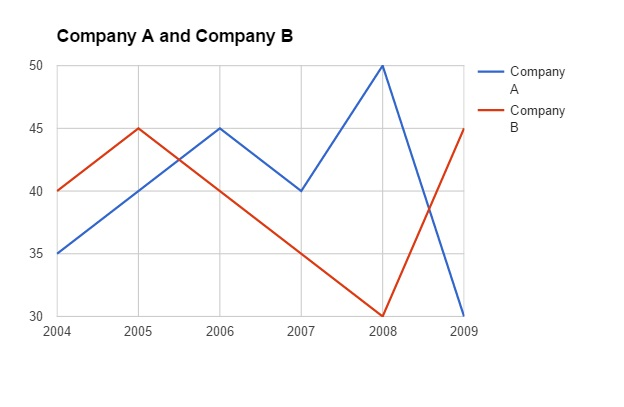# Data Interpretation Questions With Solutions For Bank Clerk

0
3660

Data Interpretation questions generally appear in the quant section in the Bank clerk and Po exams of IBPS, RBI, RRB, SBI. The topics asked from the Data Interpretation (DI) are bar graphs and pie charts. The simplification and fast calculation is the key in solving this questions, the knowledge on percentages is necessary for solving many questions on this topic.

Data Interpretation Questions With Solutions For Bank Clerk:

Instructions:

Study the graph carefully to answer the questions that follow:
Per cent profit made by two companies over the years
Per cent profit=$$\frac{Income-Expenditure}{Expenditure}\times 100$$Question 1:

If in the year 2004 the expenditures incurred by Company A and B were the same what was the ratio of the income of Company A to that company B in that year ?

a) 27:28

b) 14:23

c) 13:19

d) Cannot be determined

e) None of these

Question 2:

If the amount of profit earned by Company A in the year 2007 was Rs 1.5 lakhs what was its expenditure in that year ?

a) Rs 1.96 lakhs

b) Rs 2.64 lakhs

c) Rs 1.27 lakhs

d) Rs 3.75 lakhs

e) None of these

Question 3:

What is the average per cent profit earned by Company B over all the years together ?

a) 19

b) 24

c) 12

d) 37

e) None of these

Question 4:

If in the year 2008 the incomes of both the companies A and B were the same what was the ratio of the expenditure of Company B in that year ?

a) 21:25

b) 7:9

c) 13:15

d) Cannot be determined

e) None of these

Question 5:

What is the ratio of the amount of profit earned by Company A to that by company B in the year 2009?

a) 2:3

b) 4:7

c) 11:15

d) Cannot be determined

e) None of these

Solutions (1 to 5)

Percentage profit by company A = 35%
Percentage profit by company B = 40%
Let expenditure be x
(Income of A – x)/x = 0.35
Income of A = 1.35x
(Income of B – x)/x = 0.4
Income of B = 1.4x
Income of A:Income of B = 1.35:1.4 = 27:28

Amount of Profit = Income – Expenditure = 1.5 lakhs
Percentage of profit = 40%
1.5/x = 0.4
x = 3.75

Average percentage profit cannot be calculated because we do not know the individual income and expenditure of each year.

Percentage profits of both the companies A and B in 2008 = 50% and 30%
Let the incomes be x
(x-Expenditure of A)/Expenditure of A = 0.5
Expenditure of A = x/1.5
(x – Expenditure of B)/Expenditure of B = 0.3
Expenditure of B = x/1.3
Ratio = x/1.5:x/1.3 = 13:15